# Photoelectric Effect - JEE Advanced Previous Year Questions with Solutions

JEE Advanced Previous Year Questions of Physics with Solutions are available at eSaral. Practicing JEE Advanced Previous Year Papers Questions of Physics will help the JEE aspirants in realizing the question pattern as well as help in analyzing weak & strong areas. Get detailed Class 11th & 12th Physics Notes to prepare for Boards as well as competitive exams like IIT JEE, NEET etc. eSaral helps the students in clearing and understanding each topic in a better way. eSaral is providing complete chapter-wise notes of Class 11th and 12th both for all subjects.       Download eSaral app  for free study material and video tutorials. Simulator Previous Years JEE Advanced Questions Paragraph for Question Nos. 1 to 3 When a particle is restricted to move along x-axis between x = 0 and x = a, where a is of nanometer dimension, its energy can take only certain specific values. The allowed energies of the particle moving in such a restricted region, correspond to the formation of standing waves with nodes at its ends x = 0 and x = a. The wavelength of this standing wave is related to the linear momentum p of the particle according to the de Broglie relation. The energy of the particle of mass m is related to its linear momentum as \mathrm{E}=\frac{\mathrm{p}^{2}}{2 \mathrm{m}}. Thus, the energy of the particle can be denoted by a quantum number ‘n’ taking values 1,2,3, ... (n = l, called the ground state) corresponding to the number of loops in the standing wave. Use the model described above to answer the following three questions for a particle moving in the line x = 0 to x = a. Take h = 6.6 \times 10^{-34} \mathrm{Js} \text { and } \mathrm{e}=1.6 \times 10^{-19} \mathrm{C}.
Q. The allowed energy for the particle for a particular value of n is proportional to (A) $\mathrm{a}^{-2}$ (B) $\mathrm{a}^{-3 / 2}$ (C) $\mathrm{a}^{-1}$ $(\mathrm{D}) \mathrm{a}^{2}$ [JEE-2009]
Ans. (A)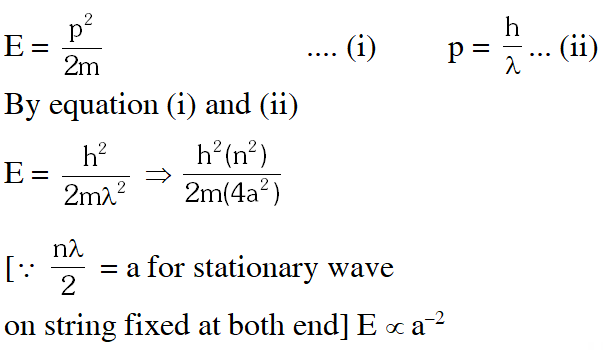Q. If the mass of the particle is m = $1.0 \times 10^{-30}$ kg and a = 6.6 nm, the energy of the particle in its ground state is closest to : (A) 0.8 meV (B) 8 meV (C) 80 meV (D) 800 meV [JEE-2009]
Ans. (B) $\mathrm{E}=\frac{\mathrm{h}^{2}}{2 \mathrm{m} 4 \mathrm{a}^{2}} \Rightarrow \frac{\left(6.6 \times 10^{-34}\right)^{2}}{2\left(1.0 \times 10^{-30}\right) \times 4 \times\left(6.6 \times 10^{-9}\right)^{2}} \frac{1}{\mathrm{e}}$ E = 8 meV
Q. The speed of the particle, that can take discrete values, is proportional to (A) $\mathrm{n}^{-3 / 2}$ $(\mathrm{B}) \mathrm{n}^{-1}$ (C) $\mathrm{n}^{1 / 2}$ (D) n [JEE-2009]
Ans. (D) $\mathrm{mv}=\frac{\mathrm{h}}{\lambda} \Rightarrow \mathrm{v}=\frac{\mathrm{h}}{\mathrm{m} \lambda} \Rightarrow \frac{\mathrm{hn}}{\mathrm{m}(2 \mathrm{a})} \Rightarrow \mathrm{v} \propto \mathrm{n}$
Q. An –particle and a proton are accelerated from rest by a potential difference of 100 V. After this, their de Broglie wavelengths are $\lambda_{\alpha}$ and $\lambda_{p}$ respectively. The ratio $\frac{\lambda_{\mathrm{p}}}{\lambda_{\alpha}}$, to the nearest integer, is ? [JEE 2010]
Ans. 3 $\frac{\mathrm{h}}{\lambda}=\mathrm{p}=\sqrt{2 \mathrm{mqV}}$ $\Rightarrow \frac{\lambda_{\mathrm{p}}}{\lambda_{\alpha}}=\sqrt{\frac{\mathrm{m}_{\alpha} \mathrm{q}_{\alpha}}{\mathrm{m}_{\mathrm{p}} \mathrm{q}_{\mathrm{p}}}}=\sqrt{4 \times 2}=\sqrt{8} \approx 3$
Q. A silver sphere of radius 1 cm and work function 4.7 eV is suspended from an insulating thread in free-space. It is under continuous illumination of 200 nm wavelength light. As photoelectrons are emitted, the sphere gets charged and acquires a potential. The maximum number of photoelectrons emitted from the sphere is $\mathrm{A} \times 10^{2}$ (where 1 < A < 10). The value of ‘Z’ is ? [JEE 2011]
Ans. 7 $\frac{h c}{\lambda}-\phi=e V=e \frac{(N e) K}{R}$ $\left(\frac{1240}{200}-4.7\right) 1.6 \times 10^{-19}=\frac{N\left(1.6 \times 10^{-19}\right)^{2} 9 \times 10^{9}}{1 / 100}$ $\frac{15}{1.6} \times 10^{7}=N$
Q. A proton is fired from very far away towards a nucleus with charge Q = 120 e, where e is the electronic charge. It makes a closest approach of 10 fm to the nucleus. The de Broglie wavelength (in units of fm) of the proton at its start is ? (Take : The proton mass, $\mathrm{m}_{\mathrm{P}}=(5 / 3) \times 10^{-27} \mathrm{kg} ; \mathrm{h} / \mathrm{e}=4.2 \times 10^{-15} \mathrm{J} . \mathrm{s} / \mathrm{C} ; \frac{1}{4 \pi \varepsilon_{0}}=9 \times 10^{9} \mathrm{m} /$ $\left.\mathrm{F} ; 1 \mathrm{fm}=10^{-15} \mathrm{m}\right)$ [JEE 2012]
Ans. 7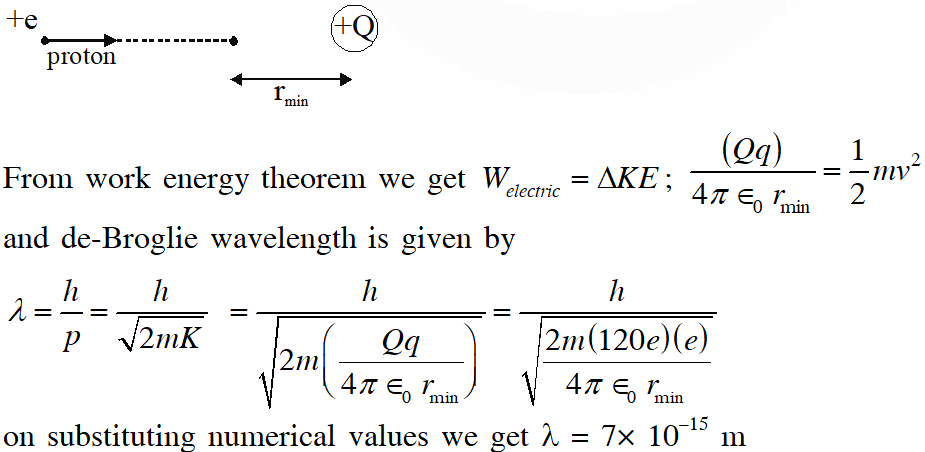Q. The work functions of Silver and sodium are 4.6 and 2.3 eV, repetitively. The ratio of the slope of the stopping potential versus frequency plot for Silver to that of Sodium is. [JEE Advanced-2013]
Ans. 1 $\mathrm{eV}_{\mathrm{s}}=\mathrm{hv}-\phi \Rightarrow \mathrm{V}_{\mathrm{s}}=\left(\frac{\mathrm{h}}{\mathrm{e}}\right) \mathrm{v}-\frac{\phi}{\mathrm{e}}$
Q. A metal surface is illuminated by light of two different wavelength 248 nm and 310 nm. The maximum speeds of the photoelectrons corresponding to these wavelengths are $\mathrm{u}_{1}$ and $\mathrm{u}_{2}$, respectively. If the ratio $\mathrm{u}_{1}: \mathrm{u}_{2}$ = 2 : 1 and hc = 1240 eV nm, the work function of the metal is nearly :- (A) 3.7 eV (B) 3.2 eV (C) 2.8 eV (D) 2.5 eV [JEE Advance-2014]
Ans. (A)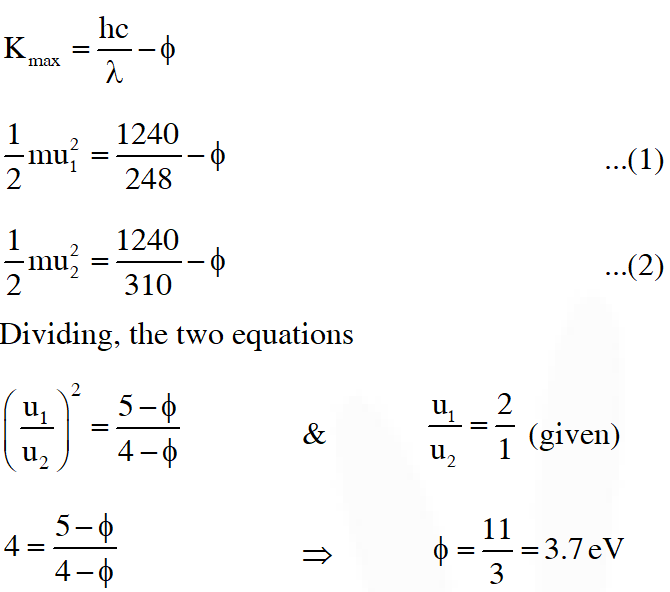Q. Planck's constant h, speed of light c and gravitational constant G are used to form a unit of length L and a unit of mass M. Then the correct option(s) is(are) :- (A) $\mathrm{M} \propto \sqrt{\mathrm{c}}$ (B) $\mathrm{M} \propto \sqrt{\mathrm{G}}$ (C) $\mathrm{L} \propto \sqrt{\mathrm{h}}$ $(\mathrm{D}) \mathrm{L} \propto \sqrt{\mathrm{G}}$ [JEE Advanced-2015]
Ans. (A,C,D)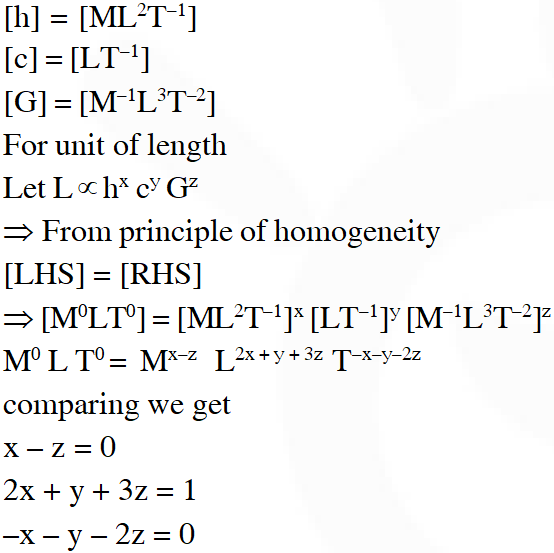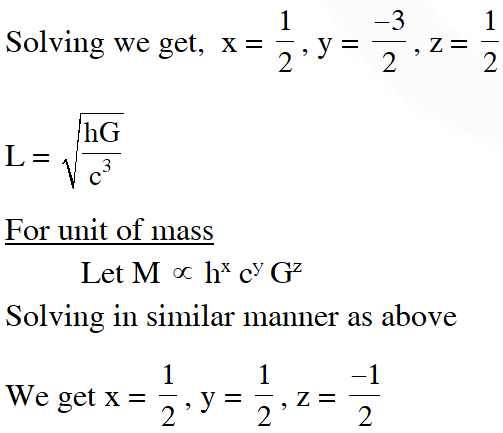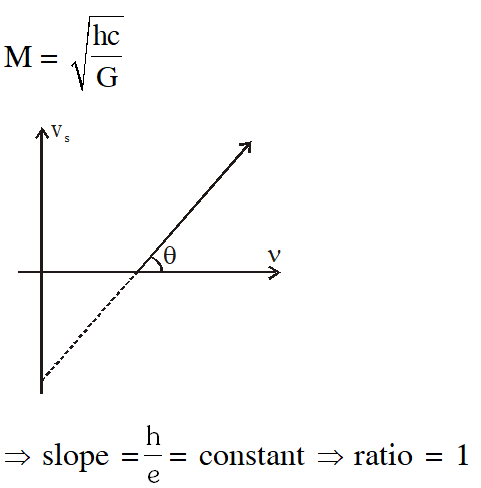Q. For photo-electric effect with incident photon wavelength $\lambda$, the stopping potential is $\mathrm{V}_{0}$. Identify the correct variation(s) of $\mathrm{V}_{0}$ with $\lambda$ and 1/$\lambda$.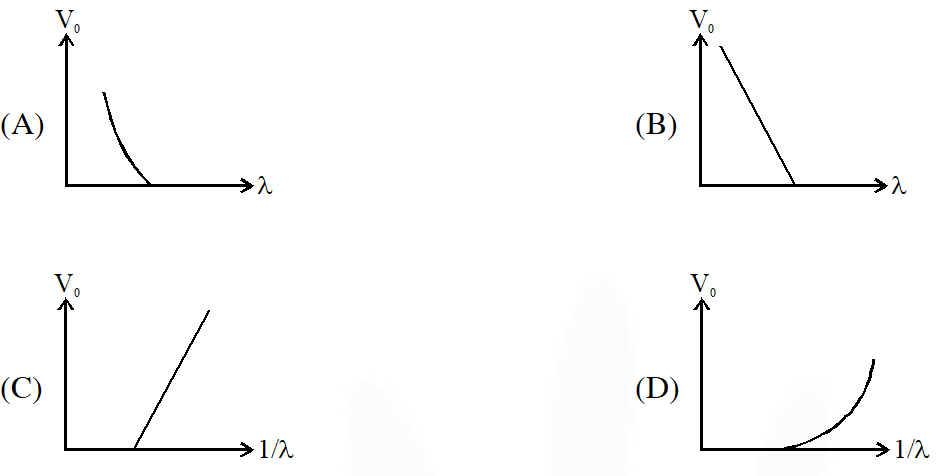[JEE Advanced-2015]
Ans. (A,C) Stopping potential $\mathrm{eV}_{0}=\frac{\mathrm{hC}}{\lambda}-\phi$ hence $\mathrm{V}_{0}$ v/s $\lambda$ curve will be hyperbola and $\mathrm{V}_{0}$ v/s $\frac{1}{\lambda}$ curve will be straight line with slope positive. hence (A,C)
Q. An electron in an excited state of $\mathrm{Li}^{2+}$ ion has angular momentum 3h/2\pi. The de Broglie wavelength of the electron in this state is p\pia, (where a is the Bohr radius). The value of $\mathrm{p}$ is? [JEE Advanced-2015]
Ans. 2 From Bohr's law $\operatorname{mvr}=\frac{\mathrm{nh}}{2 \pi}=\frac{3 \mathrm{h}}{2 \pi}(\text { from ques. })$ $\Rightarrow \mathrm{n}=3$ and momentum $=\mathrm{mv}=\frac{3 \mathrm{h}}{2 \pi \mathrm{r}}$ Now, radius of $\mathrm{n}^{\mathrm{th}}$ shell, $\mathrm{r}=\left(\frac{\mathrm{n}^{2}}{\mathrm{z}}\right) \mathrm{a}_{0}$ $\Rightarrow \mathrm{r}=\frac{(3)^{2}}{3} \cdot \mathrm{a}_{0} \quad\left[\because \mathrm{Z}_{\mathrm{Li}}=3\right]$ $\Rightarrow \mathrm{r}=3 \mathrm{a}_{0}$ wavelength $=\frac{\mathrm{h}}{\text { momentum }}$ $\Rightarrow \lambda=\frac{\mathrm{h}}{\mathrm{mv}}=\frac{\mathrm{h}}{\frac{3 \mathrm{h}}{2 \pi} \mathrm{r}}$ $\Rightarrow \lambda=\frac{2 \pi \mathrm{r}}{3}=\frac{2 \pi}{3} \times 3 \mathrm{a}_{0}$ $\lambda=2 \pi \mathrm{a}_{0}=\mathrm{p} \pi \mathrm{a}_{0}$ $\Rightarrow \mathrm{P}=2$
Q. In a historical experiment to determine Planck's constant, a metal surface was irradiated with light of different wavelengths. The emitted photoelectron energies were measured by applying a stopping potential. The relevant data for the wavelength ($\lambda$) of incident light and the corresponding stopping potential ($\mathrm{V}_{0}$) are given below: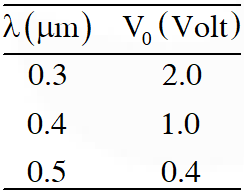Given that $c=3 \times 10^{8} \mathrm{ms}^{-1}$ and $\mathrm{e}=1.6 \times 10^{-19} \mathrm{C},$ Planck's constant (in units of $\mathrm{J}$ s) found from such an experiment is : (A) $6.0 \times 10^{-34}$ (B) $6.4 \times 10^{-34}$ (C) $6.6 \times 10^{-34}$ (D) $6.8 \times 10^{-34}$ [JEE Advanced-2016]
Ans. (B) $\mathrm{KE}_{\mathrm{max}}=\frac{\mathrm{hC}}{\lambda}-\phi$ $\mathrm{eV}_{\mathrm{s}}=\frac{\mathrm{hC}}{\lambda}-\phi$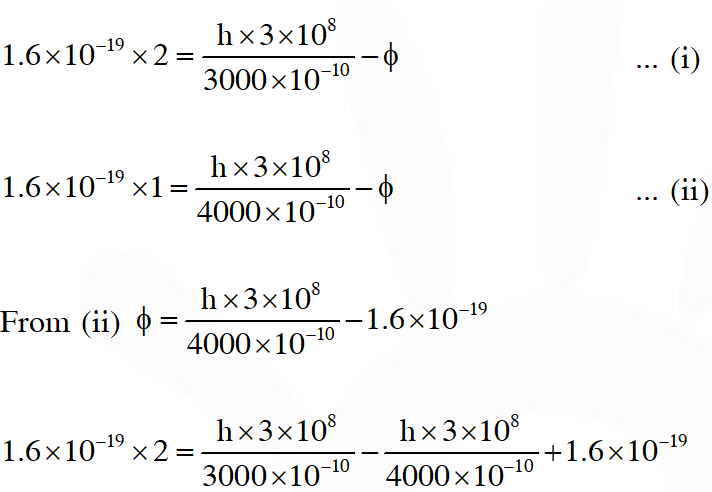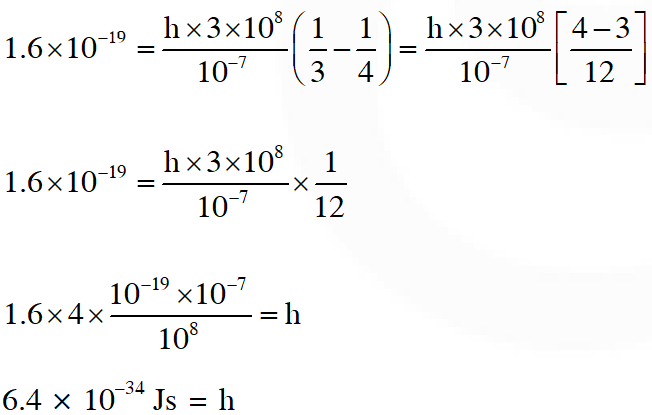Q. Light of wavelength $\lambda_{\mathrm{ph}}$ falls on a cathode plate inside a vacuum tube as shown in the figure. The work function of the cathode surface is $\phi$ and the anode is a wire mesh of conducting material kept at a distance d from the cathode. A potential difference V is maintained between the electrodes. If the minimum de Broglie wavelength of the electrons passing through the anode is $\lambda_{\mathrm{e}}$, which of the following statement(s) is(are) true ? (A) For large potential difference $(\mathrm{V}>>\phi / \mathrm{e}), \lambda_{\mathrm{e}}$ is approximately halved if $\mathrm{V}$ is made four times (B) $\lambda_{\mathrm{e}}$ increases at the same rate as $\lambda_{\mathrm{ph}}$ for $\lambda_{\mathrm{ph}}<\mathrm{hc} / \phi$ (C) $\lambda_{\mathrm{e}}$ is approximately halved, if d is doubled (D) $\lambda_{\mathrm{e}}$ decreases with increase in $\phi$ and $\lambda_{\mathrm{ph}}$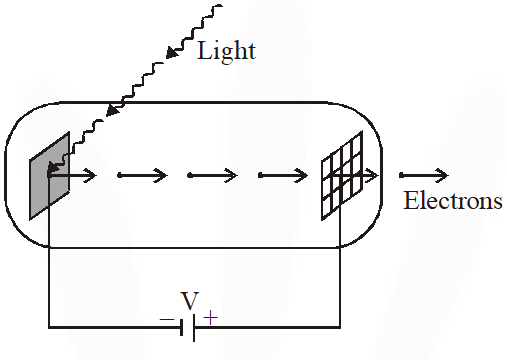[JEE Advanced-2016]
Ans. (A) $\mathrm{K}_{\max }=\frac{\mathrm{hc}}{\lambda_{\mathrm{Ph}}}-\phi$ kinetic energy of $\mathrm{e}^{-}$ reaching the anode will be $\mathrm{K}=\frac{\mathrm{hc}}{\lambda_{\mathrm{Ph}}}-\phi+\mathrm{eV}$ Now $\lambda_{\mathrm{e}}=\frac{\mathrm{h}}{\sqrt{2 \mathrm{mK}}}=\frac{\mathrm{h}}{\sqrt{2 \mathrm{m}\left(\frac{\mathrm{hc}}{\lambda_{\mathrm{Ph}}}-\phi+\mathrm{eV}\right)}}$ If $\mathrm{eV}>>\phi$ $\lambda_{e}=\frac{\mathrm{h}}{\sqrt{2 \mathrm{m}\left(\frac{\mathrm{hc}}{\lambda_{\mathrm{ph}}}+\mathrm{eV}\right)}}$ If $\mathrm{V}_{\mathrm{f}}=4 \mathrm{V}_{\mathrm{i}}$Q. A photoelectric material having work-function $\phi_{0}$ is illuminated with light of wavelength $\lambda$ $\left(\lambda<\frac{\mathrm{hc}}{\phi_{0}}\right) .$ The fastest photoelectron has a de-Broglie wavelength $\lambda_{\mathrm{d}}$. A change in wavelength of the incident light by $\Delta \lambda$ results in a change $\Delta \lambda_{\mathrm{d}}$ in $\lambda_{\mathrm{d}} .$ Then the ratio $\Delta \lambda_{\mathrm{d}} / \Delta \lambda$ is proportional to :- $(A) \lambda_{d}^{3} / \lambda^{2}$ (B) $\lambda_{\mathrm{d}}^{3} / \lambda$ (C) $\lambda_{\mathrm{d}}^{2} / \lambda^{2}$ (D) $\lambda_{d} / \lambda$ (A) According to photo electric effect equation : $\mathrm{KE}_{\max }=\frac{\mathrm{hc}}{\lambda}-\phi_{0}$ $\frac{\mathrm{p}^{2}}{2 \mathrm{m}}=\frac{\mathrm{hc}}{\lambda}-\phi_{0}$ $\frac{\left(\mathrm{h} / \lambda_{\mathrm{d}}\right)^{2}}{2 \mathrm{m}}=\frac{\mathrm{hc}}{\lambda}-\phi_{0}$ Assuming small changes, differentiating both sides, $\frac{\mathrm{h}^{2}}{2 \mathrm{m}}\left(-\frac{2 \mathrm{d} \lambda_{\mathrm{d}}}{\lambda_{\mathrm{d}}^{3}}\right)=-\frac{\mathrm{hc}}{\lambda^{2}} \mathrm{d} \lambda$ $\frac{\mathrm{d} \lambda_{\mathrm{d}}}{\mathrm{d} \lambda} \propto \frac{\lambda_{\mathrm{d}}^{3}}{\lambda^{2}}$
Ans.

Sachin
Sept. 2, 2021, 8:07 p.m.